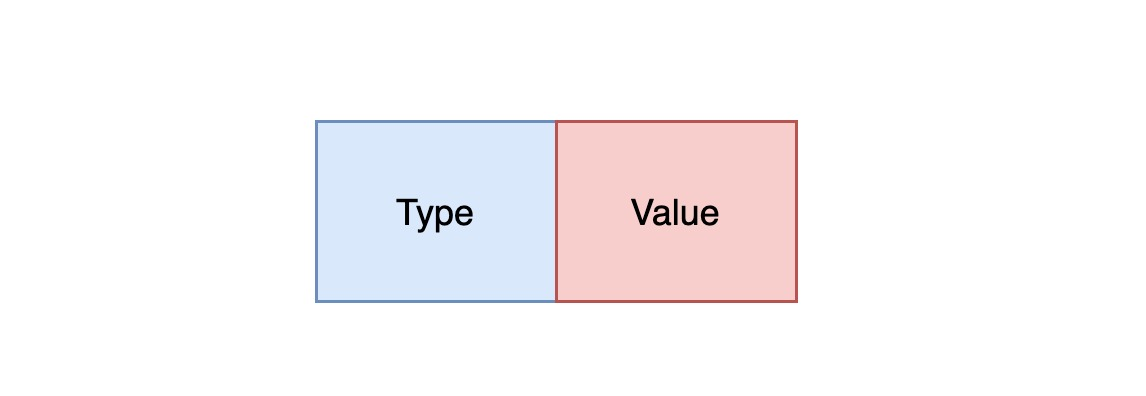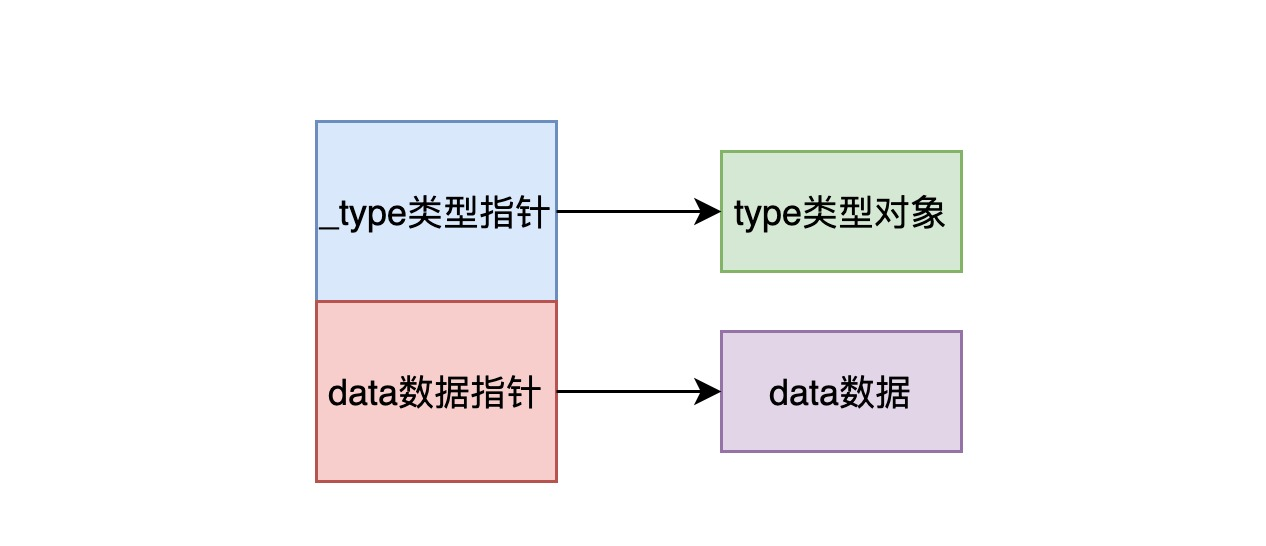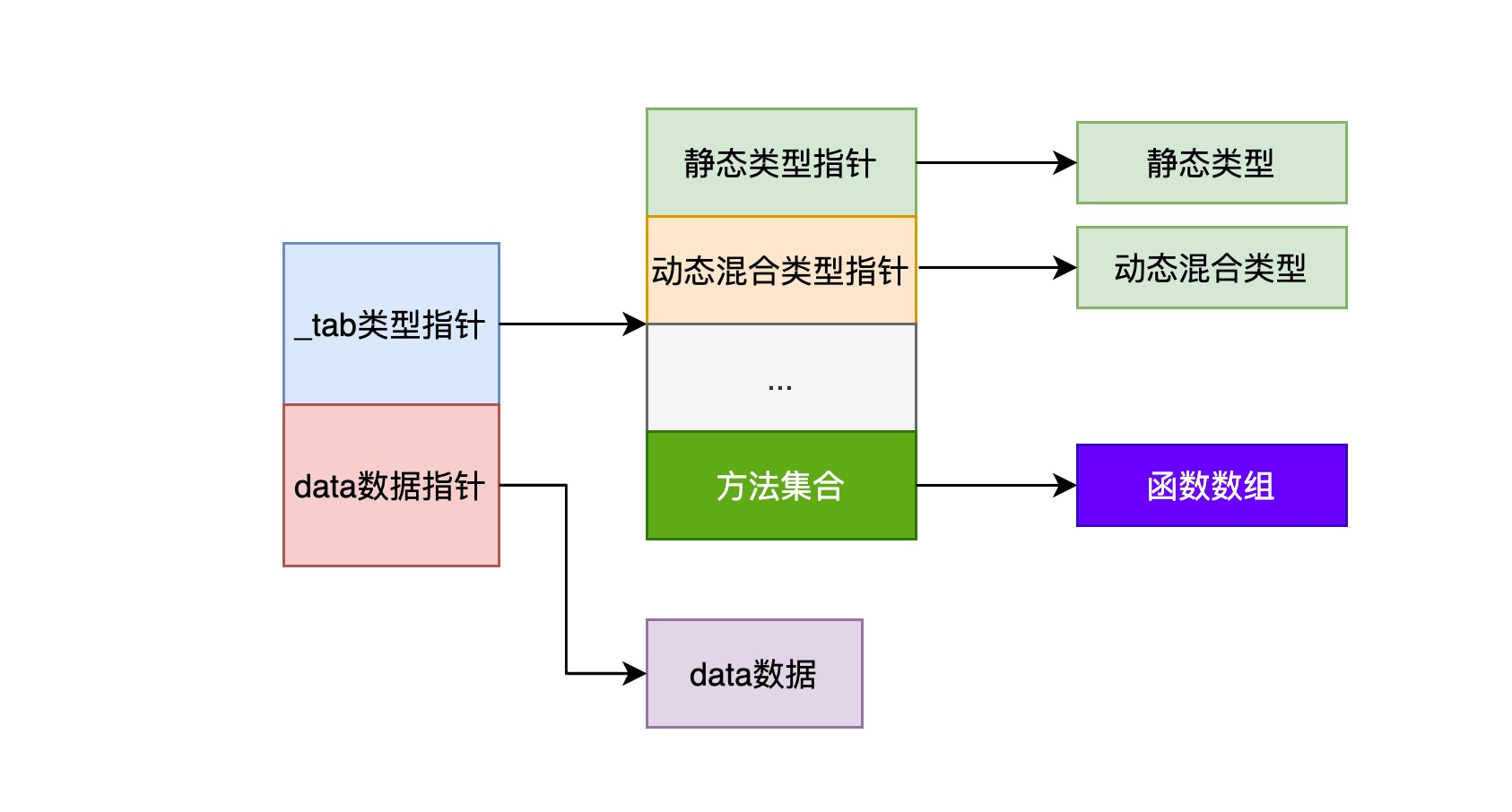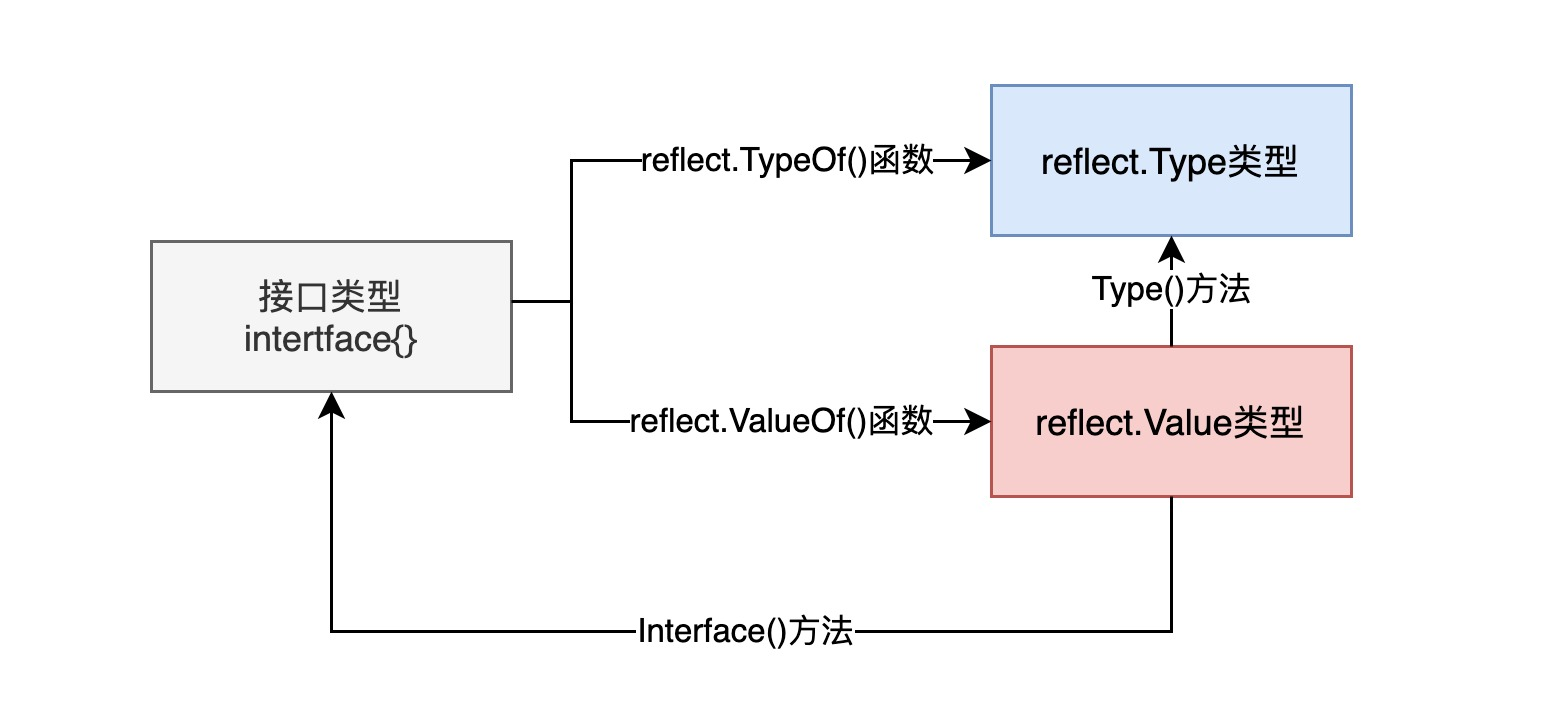Many languages support reflection, and the Go language is no exception. So what is reflection? In a nutshell, reflection is the ability of a computer programming language to dynamically access, inspect, and modify the state and behavior of any object itself at runtime. Reflection features work differently in different languages, and some languages do not support reflection features. Today we’ll focus on how reflection works in Go.

We recommend you read the official Go blog on Reflection: The Laws of Reflection

## Scenarios for reflection

It is important to note that Go is a statically typed compiled language, which means that the compiler can find some type errors during compilation, but it cannot find errors in reflection-related code, which often need to be found at runtime, so if there is an error in the reflection code, it will directly cause the program to panic and exit. In addition, reflection-related code is often less readable and less efficient than normal Go code. In summary, we should avoid using Go’s reflection features unless we have to use them in the following special cases.

Generally speaking, the scenarios that apply to reflection include the following.

• The data type to be processed by a function or method is uncertain and will contain some columns of possible types, so we need to use reflection to dynamically obtain the data type to be processed and call different handlers based on the data type. A very typical example is to get a data structure of type `map[string]interface{}` by deserialization, and then get the type of each internal field recursively by reflection.
• In some scenarios we need to decide which function to call based on specific conditions, that is, we need to get the function and the parameters required for the function to run during runtime to call the function dynamically. A typical example is the implementation mechanism of the mainstream RPC framework, where the RPC server maintains a mapping of function names to function reflections, and the RPC client passes function names and parameter lists to the RPC server over the network, which is then parsed by the RPC server as reflections to invoke and execute the function, and finally the return value of the function is packaged and returned to the RPC client over the network.

Note: Of course, there may be other application scenarios that apply to reflection, so this is just a list of common usage scenarios.

## The Basics of Reflection

The first thing that needs to be made clear is that the basis of Go reflection is type. In Go, each variable has a static type, which is the type that needs to be checked at the compilation stage, such as `int`, `string`, `map`, `struct`, and so on. Note that this static type is the type specified when the variable is declared, and not necessarily the type of data actually stored at the bottom.

For example.

 ``````1 2 3 4 `````` ``````type MyInt int var i int var j MyInt ``````

In the above code, although the variables `i` and `j` are both really stored with the data type `int`, they are different types to the compiler and have to undergo a display type conversion to assign values to each other.

Some variables may have dynamic types in addition to static types. A dynamic type is information about the type of variable value actually stored, for example.

 `````` 1 2 3 4 5 6 7 8 9 10 11 `````` ``````var r io.Reader tty, err := os.OpenFile("/dev/tty", os.O_RDWR, 0) if err != nil { return nil, err } r = tty var empty interface{} empty = tty ``````

The first statement declares `r` to be of type `io.Reader`, which means that it is a static type of `r`, its dynamic type is `nil`, and its dynamic value is also `nil`. The next `r=tty` statement changes the dynamic type of `r` to `*os.File` and the dynamic value to non-null, indicating an open file object. At this point, `r` can be represented by a `<value,type>` pair as `<tty, *os.File>`.

Also, we know that any object in the Go language is of type empty interface (`interface{}`), because any object implements zero or more methods. So the statement `empty = tty` assigns the `*os.File` object to the variable `empty` of type empty interface.

In fact, reflection is closely related to the null interface type. any object of type in the Go language is composed of two main parts.

1. Type
2. ValueIn fact, if you want to break it down, you can divide it into `eface` without function and `iface` with function.

1. functionless `eface`2. `iface` with function`iface` describes a non-empty interface, which contains the set of methods of that type; in contrast, `eface` describes an empty interface (`interface{}`), which does not contain any methods.

## Basic types and functions for reflection

The `reflect` standard library defines two important types of reflection objects, corresponding to the two components of an object of any of the Go language types described earlier.

1. `reflect.Type`
2. `reflect.Value`

These two reflection object types provide a number of functions to get the types stored in the interface. One of them, `reflect.Type`, is defined as an interface type and provides mainly information about the type. From the definition of the `Type` interface, you can see many interesting methods, including `MethodByName` to get the reference of the method corresponding to the current type, `Implements` to determine whether the current type implements an interface, etc.

 `````` 1 2 3 4 5 6 7 8 9 10 11 12 13 14 `````` ``````type Type interface { Align() int FieldAlign() int Method(int) Method MethodByName(string) (Method, bool) NumMethod() int Name() string PkgPath() string Size() uintptr String() string Kind() Kind Implements(u Type) bool ... } ``````

In contrast, `reflect.Value` is a normal structure without any externally exposed member variables, but it provides many ways to get or write data stored in the `reflect.Value` structure, so you can get or even change the value of the type through it.

 ``````1 2 3 4 5 6 7 8 9 `````` ``````type Value struct { // contains filtered or unexported fields } func (v Value) Addr() Value func (v Value) Bool() bool func (v Value) Bytes() []byte func (v Value) Float() float64 ... ``````

Also, the `reflect` standard library provides two basic reflection functions to get these two reflection type objects.

1. `func TypeOf(i interface{}) Type`
2. `func ValueOf(i interface{}) Value`

The `TypeOf` function is used to extract the type information of a value in an interface. Since its input parameter is an empty interface (`interface{}`), when this function is called, the real parameter is first converted to the empty interface type; the `ValueOf` function also accepts an empty interface, so the real parameter also has to be type converted. The return value of the function is `reflect.Value` which indicates the value of the actual variable stored in the parameter value, and it provides various information about the actual variable, such as the type structure pointer, the address of the real data, the flag bit, etc.

In particular, it should be noted that the `reflect.Value` reflected object value obtained by the `ValueOf` function has the following two important methods.

1. the `Type()` method gets the type information of the variable, which is equivalent to calling the `reflect.TypeOf()` function directly with the interface type.
2. the `Interface()` method gets information about the interface type of the reflected variable, which is equivalent to the reverse of the `ValueOf` function.## The Three Laws of Reflection

According to the official Go blog, the three laws of reflection are summarized as follows.

1. Reflection goes from interface value to reflection object.
2. Reflection goes from reflection object to interface value.
3. To modify a reflection object, the value must be settable.

The first law of reflection says that based on reflection we can convert interface type objects into reflection type objects and thus detect the types and values stored in the interface type objects, the `reflect.TypeOf` and `reflect.ValueOf` mentioned above are two important functions for this conversion.

As an example.

 ``````1 2 3 4 5 6 7 `````` ``````var x float64 = 3.4 t := reflect.TypeOf(x) v := reflect.ValueOf(x) fmt.Println("type:", t) fmt.Println("type:", v.Type()) fmt.Println("kind is float64:", v.Kind() == reflect.Float64) fmt.Println("value:", v.Float()) ``````

The output is as follows.

 ``````1 2 3 4 `````` ``````type: float64 type: float64 kind is float64: true value: 3.4 ``````

The above simple example illustrates how we can transform an interface type variable `x` into a reflection type object `t` and `v` with the `reflect.TypeOf` and `reflect.ValueOf` functions, and thereby obtain other meta-information about the variable, including data and operations related to the current type, for example, through the `Kind()` method to obtain the reflection object is stored as a base type, etc.

• For structures, you can get the number of fields and get the individual fields by index and field name.
• For a hash table, you can get the key values of the hash table.
• For functions or methods, you can get the types of the input and return values.

The second law of reflection is the opposite of the first law, in that reflected objects can also be reduced to variables of interface type. Objects of type `reflect.Value` can obtain interface variables of type `interface{}` by calling the `Interface()` method.

 ``````1 2 `````` ``````v := reflect.ValueOf(1) v.Interface{}.(int) ``````

As you can see from the above example, the process of converting a reflected object to an interface object is like mirroring the process from an interface object to a reflected object. From an interface object to a reflected object, you need to go through a type conversion from the basic type to the interface type and from the interface type to the reflected object type, and in turn, the reflected object needs to be converted to an interface type first, and then to the original type through forced type conversion.

The third law of reflection relates to whether a value can be changed. It says that if a reflected variable needs to be modified, then it must be settable. The essence of a reflective variable being settable is that it stores the original variable itself, so that an operation on the reflective variable will be reflected in the original variable itself; conversely, if the reflective variable does not represent the original variable, then an operation on the reflective variable will not have any effect on the original variable, which can cause confusion to the user. So the second case is forbidden at the language level.

As an example.

 ``````1 2 3 4 5 6 7 `````` ``````\$ cat << EOF > reflect.go > var x float64 = 3.4 > v := reflect.ValueOf(x) > v.SetFloat(7.1) // Error: will panic. > EOF \$ go run reflect.go panic: reflect: reflect.flag.mustBeAssignable using unaddressable value ``````

The above code will panic because the reflection variable `v` does not represent the reflection object of the interface variable `x` itself, because the Go language function call parameter passing is value passing, so the function `reflect.ValueOf(x)` is executed with a copy of the formal reference, so `v` is not a true reflection object map of `x`. Therefore, the Go language prohibits operations on `v`.

Settable is a property of the reflection object `reflect.Value`, but not all reflection objects are settable. If we want the above example to work, we just need to pass a pointer to the argument when the function is called and it will work out.

 ``````1 2 3 4 `````` ``````var x float64 = 3.4 p := reflect.ValueOf(&x) fmt.Println("type of p:", p.Type()) fmt.Println("settability of p:", p.CanSet()) ``````

However, although the program can run, the output shows that the reflection object obtained by the pointer is still “unsettable”.

 ``````1 2 `````` ``````type of p: *float64 settability of p: false ``````

The reason is that the reflection object `p` does not yet represent `x`. `p` is a pointer type inside the reflection object, and the real type it points to needs to be obtained from `p.Elem()`, i.e. `p.Elem()` can only represent `x`.

 ``````1 2 3 4 `````` ``````v := p.Elem() v.SetFloat(7.1) fmt.Println(v.Interface()) // 7.1 fmt.Println(x) // 7.1 ``````

In short, if you want to manipulate the original variable, the reflected variable `Value` must correspond to the address of the original interface variable.

## Summary

Reflection is one of the more important features of Go, and many frameworks rely on Go’s reflection mechanism to implement some dynamic features.

As a static language, Go is designed to be as simple as possible, but sometimes lacks expressiveness. The good thing is that Go provides dynamic features to compensate for its syntactic disadvantages through the reflection mechanism.

This article provides a general understanding of the use of reflection in Go, the basic implementation mechanism of reflection, and introduces the basic types and functions of reflection and the Three Laws. On the whole, Go reflection revolves around three types, `Type`, `Value` and `Interface{}`, which are complementary to each other. The `reflect` standard library implements runtime reflection capabilities that allow Go programs to manipulate objects of different types, using the `reflect.TypeOf` function to obtain dynamic type information from static interface types and the `reflect.ValueOf()` function to obtain a runtime representation of the data, using these two functions and the `reflect` other methods of the standard library to get a more powerful representation.